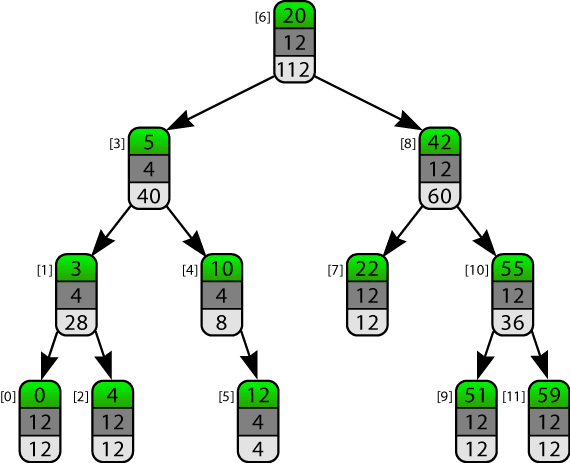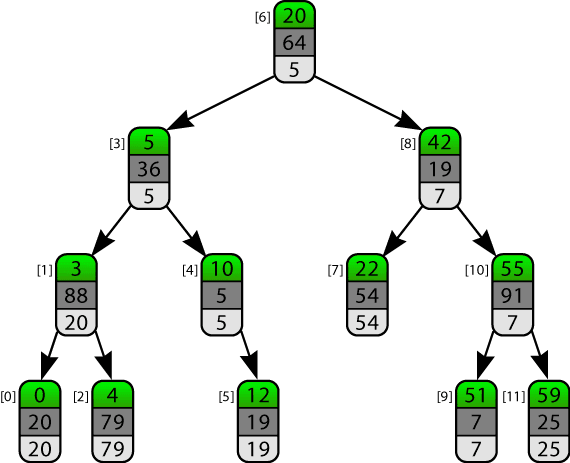### Boost.Intrusive Annotated Trees: Part 1

Most programming problems can be solved using only standard, well-known data structures. Occasionally, though, there's a problem that needs something special.

I needed a container for a GUI app that would display a large, sorted, scrollable table of data, whilst receiving constant updates to the data being displayed. The result is a set of extensions to boost.intrusive that let you create custom extensions to tree-based data strucutres to do things elegantly that would otherwise be difficult, tedious, inefficient or some combination thereof.

## The Problem

To process updates to the table, efficient removal and insertion of items is a must. This is the natural habitat of the balanced binary trees, e.g. the ever-popular red/black tree - both operations are O(log n) worst-case. So far so good.

To draw the visible part of the table, I'd calculate what row numbers should be visible based on the scrollbar position, then retrieve those rows from the container. That's a problem for binary trees, because finding the nth element in binary trees is linear time.

The only standard container that has better-than-linear indexing is an array - but arrays have O(n) insertion/removal, and there's nothing you can do to improve on that. So arrays are out of the running - but there's still hope for the tree-based approach.

## The tree with fast indexing

Everyone loves pictures, so here's a plain binary tree to get us started:

It's just a simple sorted binary tree containing the integers `[0, 3, 4, 5, 10, 12, 20, 22, 42, 51, 55, 59]`. For this method of indexing to be logarithmic time, it has to be some form of balanced binary tree (e.g. red-black, AVL), but it doesn't really matter which.

I've also labelled each tree node with its position in the container - the bracketed numbers. These indexes are not part of the data structure, they're just on the diagram to help the discussion.

As it stands, to find the nth node in this tree, we have to start at the leftmost node and walk the tree depth-first until we've visited n nodes. However, by storing one extra number at each node, we can make it possible to find the nth node in logarithmic time.

The number we need to store is the total number of nodes in the sub-tree below it (its sub-tree size). When we store the sub-tree size as part of the node, we are annotating the node with its own sub-tree size.

Here's what the tree looks like with the sub-tree size annotation:

To calculate and maintain these values, we need to modify the insertion and removal algorithms for our tree. For example, if we were to insert the value '63' into this container, we'd first walk down the tree to find the position where it belongs, and then link it in. Let's say our insertion algorithm decides to make it the right child of node .

To maintain the integrity of our sub-tree size annotation, we then need to iterate back up the tree re-calculating the annotation value for all values between the newly inserted node and the root: node 's annotation would become 2, node 's annotation would become 4, node 's annotation would become 6, and the root node's annotation would become 13.

At each node all we're calculating is (left child's annotation + 1 + right child's annotation). This is a constant-time operation, applied to a log(n) nodes, so the overall insertion operation is still logarithmic. Removing nodes is a bit more complicated, and node rotations for re-balancing also need to be taken into account, but the details aren't all that interesting.

Now that we've got our tree with sub-tree sizes, we can use them to tell whether the nth element of the sub-tree starting at any given node is in under the left or right child of that node, giving us a logarithmic time indexing operation. For more detail on how this works, see the appendix below.

## The generalisation

Having logarithmic-time indexing facilitated by a sub-tree size annotation is all well and good, but there's a lot more you can do with annotations. Earlier, I said that the GUI application would calculate what row numbers needed to be drawn on-screen based on the scrollbar position. Unfortunately, that only works if all rows are the same height. If our rows are resizeable, we can't even find out what row numbers should be drawn on-screen without a linear-time operation, so fast indexing doesn't help at all.

What we need instead is a way of finding out what row occupies the space n pixels from the top of the data table. In our sub-tree size annotation, each node had an implicit value of one — one node per node. Let's change that so each item's own value is instead its height in pixels. I'll call this the input value for the annotation.Input value: pixel height, Annotation: (left + input + right)

Most of our rows are 12 pixels high, but rows , ,  and  have been adjusted to be just 4 pixels high. These are the input values for the annotation, and they can been seen in the middle box (dark grey background) of each node in the diagram above.

The calculation used for the annotation is now (left child's annotation + input value + right child's annotation). Previously the annotation held a sub-tree count, now it's the sum of the pixel heights of all the nodes in the sub-tree.

Using this annotation, we can find the row that occupies the space n pixels from the top of data table in logarithmic time, in much the same way that we used for finding the nth row using sub-tree counts.

What else? Let's suppose each of our rows has an associated priority, and we need to find the highest priority in logarithmic time (note: higher numbers mean lower priority). Let's make the input value the node's priority, and change the calculation to be (minimum(left child's annotation, input value, right child's annotation))Input value: priority, Annotation: min(left, input, right)

The highest priority is item  with priority 5, and the annotations show the highest priority item of any node in the sub-tree rooted at that node.

Ta-dah, it's a priority queue! It's not a great priority queue; finding the highest-priority item in it is O(log n), not O(1). However, if you've got to have the unannotated data structure anyway then this can be more space-efficient.

## The rub

It all sounds great so far — we can add bespoke functionality to a red-black tree without changing the complexity of most of the important operations: insertion is still O(log n), and removal by key is still O(log n).

Unfortunately, it's not a free ride all the way. Hinted insertion in red-black trees is amortized constant time, but with annotations it becomes O(log n). Removing nodes is also amortized constant time when you specify the node to be removed by iterator or node reference, but like insertion it becomes O(log n) with annotations.

There are some annotations where, under certain conditions, these two operations will still be amortized constant time, but they are the exception rather than the rule. An example is the maximum-priority annotation, where hinted insertion and deletion are still amortised constant time if the insertions/deletions are randomly ordered. Proof of this is left as an exercise for the reader.

## The code

These ideas aren't new. There's a great article by Mark Chu-Caroll on purely functional data structures that discusses using this approach with an external data structure rather than integrating it into a container. Mark also discusses how all the annotations I've shown so far can be described as monoids (although actually you only need a semigroup). Allan Odgaard has described using this approach for a text editor's layout, and Simon Tatham has a page on applying this to B-Trees

What is new, however, is that you don't have to write your own data structure to use them any more.

I've been working on extending the Boost.Intrusive container library to support arbitrary user-specified annotations, and the work in progress is available on github. Currently `boost::intrusive::rbtree` is the only container with annotations support, but that's purely because of the order I've chosen to do things in.

So, how do you define and use your own annotations with Boost.Intrusive Annotated Trees? Unfortunately, I'm not going to delve into that here and now. That'll be the subject of a future blog post, but here's a teaser:

```#include <boost/intrusive/semigroup_annotation.hpp>
#include <boost/intrusive/set_hook.hpp>
#include <boost/intrusive/rbtree.hpp>
#include <iostream>

using namespace boost::intrusive;

struct my_annotation : fixed_value_semigroup_annotation<
my_annotation, std::size_t, std::plus<std::size_t>, 1>
{};

struct TreeNode : public set_base_hook<annotations<my_annotation> >
{ int val; };

bool operator<(const TreeNode& a, const TreeNode& b)
{ return a.val < b.val; }

int main() {
TreeNode nodes;
rbtree<TreeNode> tree;
for(auto& n: nodes)
tree.insert_equal(n);
for(auto& n: nodes)
std::cout << n.get_annotation_value<my_annotation>()
<< std::endl;
return 0;
}
```

† except for complete binary trees, which don't have O(log n) insertion/removal

#### 2 comments

1.I was looking for something like this recently...

2.Great. I was also looking at something like this.